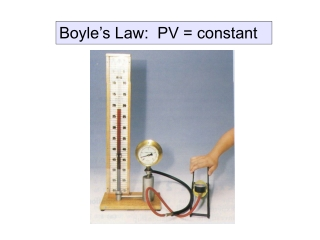DownloadDownload PresentationBoyle’s Law: PV = constant

# Boyle’s Law: PV = constant

Télécharger la présentation## Boyle’s Law: PV = constant

- - - - - - - - - - - - - - - - - - - - - - - - - - - E N D - - - - - - - - - - - - - - - - - - - - - - - - - - -
##### Presentation Transcript

1. Boyle’s Law: PV = constant

2. Pressure Law: P/T = constant

3. Charles’ Law: V/T = constant

4. General Gas Equation Boyle’s Law: PV = constant Charles’ Law: V/T = constant Pressure Law: P/T = constant PV/T = constant

5. PV/T = constant So, P1V1/T1 = P2V2/T2

6. Solution: P1V1/T1 = P2V2/T2 PV/T = 2P2V/T2 T2 = 4T

7. Solution: P1V1/T1 = P2V2/T2 3x105 (V) / (273+30) = P2 (2V) / (273+60) P2 = 1.65 x105 Pa

8. Solution: P1V1/T1 = P2V2/T2 P V / T = 2P (V2) / 0.5T V2 = 0.25 V